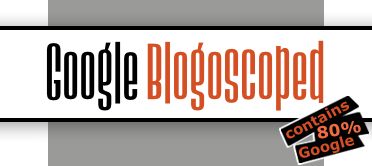### Wednesday, January 31, 2007

Here's what you need to do to make Google go crazy (this supposedly works for other websites too, though I only got it to work with Google):

2. Remove everything from the address bar, then copy & paste the following into it:

javascript:R=0; x1=.1; y1=.05; x2=.25; y2=.24; x3=1.6; y3=.24; x4=300; y4=200; x5=300; y5=200; DI=document.images; DIL=DI.length; function A(){for(i=0; i-DIL; i++){DIS=DI[ i ].style; DIS.position='absolute'; DIS.left=Math.sin(R*x1+i*x2+x3)*x4+x5; DIS.top=Math.cos(R*y1+i*y2+y3)*y4+y5}R++}setInterval('A()',5); void(0);
3. Hit enter. Enjoy.

[Via Digg. Thanks Suresh S.!]